Tamilnadu State Board New Syllabus Samacheer Kalvi 11th Maths Guide Pdf Chapter 4 Combinatorics and Mathematical Induction Ex 4.2 Text Book Back Questions and Answers, Notes.

## Tamilnadu Samacheer Kalvi 11th Maths Solutions Chapter 4 Combinatorics and Mathematical Induction Ex 4.2

Question 1.
If (n – 1)P3 : nP4 = 1 : 10 find n.
Given (n – 1)P3 : nP4 = 1 : 10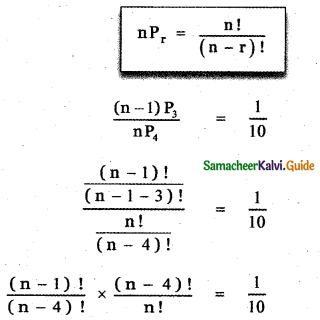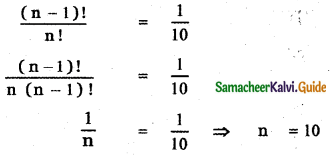Question 2.
If 10Pr – 1 = 2 × 6Pr, find r.
Given 10Pr – 1 = 2 × 6Pr5 × 9 × 8 × 7 = (11 – r) (10 – r) (9 – r) (8 – r) (7 – r)
5 × 3 × 3 × 2 × 4 × 7 = (11 – r) (10 – r) (9 – r) (8 – r)(7 – r)
7 × 6 × 5 × 4 × 3 = ( 11 – r ) (10 – r) (9 – r) (8 – r) (7 – r)
(11 – 4) (10 – 4) (9 – 4) (8 – 4) (7 – 4)
= (11 – r) (10 – r) (9 – r) (8 – r) (7 – r)
∴ r = 4Question 3.
(i) Suppose 8 people enter an event in a swimming meet. In how many ways could the gold, silver and bronze prizes be awarded?
From 8 persons we have to select and arrange 3 which can be done in 8P3 ways So the prizes can be awarded in 8P3 = 8 × 7 × 6 = 336 ways

(ii) Three men have 4 mats, 5 waist coats and 6 caps.In how many ways can they wear them?
Number of men = 3
Number of coats = 4
Number of waist coats = 5
Number of caps = 6
4 coats can be given to 3 men in 4P3 ways.
5 waist coats can be given to 3 men in 5P3 ways.
6 caps can be given to 3 men in 6P3 ways.
∴ Total number of ways of wearing 3 coats, 4 waist coats and 6 caps is
= 4P3 × 5P3 × 6P3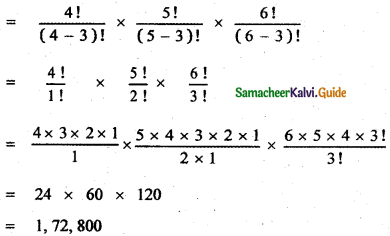= 1, 72, 800Question 4.
Determine the number of permutations of the letters of the word SIMPLE If all are taken at a time?
SIMPLE
Total Number of letters = 6
They can be arranged in 6! ways
∴ The number of words = 6! = 6 × 5 × 4 × 3 × 2 × 1 = 720

Question 5.
A test consists of 10 multiple choice questions. In how many ways can the test be answered if
(i) Each question has four choices ?
(ii) The first four questions have three choices and the remaining have five choices?
(iii) Question number n has n + 1 choices ?
Each question has 4 choices. So each question can be answered in 4 ways.
Number of Questions = 10
So they can be answered in 410 ways

(ii) The first four questions have 3 choices. So they can be answered in 34 ways.
The remaining 6 questions have 5 choices. So they can be answered in 56 ways.
So all 10 questions can be answered in 34 × 56 ways.

(iii) Given question n has n + 1 choices
question 1 has 1 + 1 = 2 choices
question 2 has 2 + 1 = 3 choices
question 3 has 3 + 1 = 4 choices
question 4 has 4 + 1 = 5 choices
question 5 has 5 + 1 = 6 choices
question 6 has 6 + 1 = 7 choices
question 7 has 7 + 1 = 8 choices
question 8 has 8 + 1 = 9 choices
question 9 has 9 + 1 = 10 choices
So the number of ways of answering all the 10 questions = 2 × 3 × 4 ×…. × 11 = 11! waysQuestion 6.
A student appears in an objective test which contain 5 multiple choice, questions. Each question has 4 choices, out of which one correct answer.
(i) What is the maximum number of different answers can the students give?
(ii) How,will the answer change if each question may have more than one correct answer ?
(i) What is the maximum number of different answers can the students give?
Selecting a correct answer from the 4 answers can be done in 4 ways.
Total number of questions = 5 So they can be answered in 45 ways

(ii) How will the answer change if each question may have more than one correct answers? Since each question may have more than one correct answer, each question can have the possibilities 1, 2, 3 or 4 correct answers.
∴ Number of ways of answering each question
= 4C1 + 4C2 + 4C3 + 4C4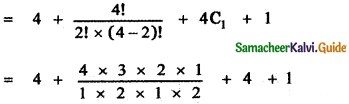= 4 + 6 + 4 + 1 = 15
Thus, the answer will change as 155 (i.e, Total number of ways of answering five questions).

Question 7.
How many strings can be formed from the letters of the word ARTICLE, so that vowels occupy even places?
ARTICLE
Vowels A, I, E = 3
Total number of places = 7
1 2 3 4 5 6 7
Number of even places = 3
3 Vowels can occupy 3 places in 3! = 3 × 2 × 1 = 6 ways
Then the remaining 4 letters can be arranged in 4! ways
So total number of arrangement = 3! × 4! = 6 × 24 = 144 waysQuestion 8.
8 women and 6 men are standing in a line.
(i) How many arrangements are possible if any individual can stand in any position ?
(ii) In how many arrangements will all 6 men be standing next to one another?
(iii) In how many arrangements will no two men be standing next to one another?
Number of women standing in a line = 8
Number of men standing in a line = 6

(i) How many arrangements are possible if any individual can stand In any position?
Total number of persons = 8 + 6 = 14
They can be arranged in 14! ways

(ii) In how many arrangements will all 6 men be standing next to one another?
There are 6 men and 8 women. To make all 6 men together treat them as 1 unit. Now there are 1 + 8 = 9 persons.
They can be arranged in 9! ways. After this arrangement, the 6 men can be arranged in 6! ways.
So total number of arrangements = 9! × 6!

(iii) In how many arrangements will no two men be standing next to one another?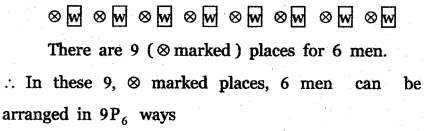8 women placed in the box can be arranged in 8P8 = 8! ways
∴ Total number of ways = 9P6 × 8!

Question 9.
Find the distinct permutations of the letters of the word MISSISSIPPI.
The given word is MISSISSIPPI.
Number of letters in the word = 11
Number of S’s = 4
Number of I’s = 4
Number of P’s = 2
Number of M’s = I
Hence the total number of distinct words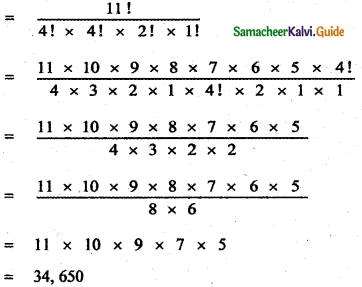Question 10.
How many ways can the product a2 b3 c4 be expressed without exponents?
The given term is a2b3c4
The factors are a, b, c
Number of a’s = 2
Number of b’s = 3
Number of c’s = 4
a2b3c4 = a×a × b×b×b × c×c×c×c
Total number of factors in the product = 9
Number of ways the product can be expressed without exponentsQuestion 11.
In how many ways 4 mathematics books, 3 physics books, 2 chemistry books and 1 biology book can be arranged on a shelf so that all books of the same subjects are together:
Number of maths book = 4
Number of physics books = 3
Number of chemistry books = 2
Number of biology books = 1
Since we want books of the same subjects together, we have to treat all maths books as 1 unit, all physics books as 1 unit, all chemistry books as 1 unit, and all biology books as 1 unit.
Now the total number of units = 4
They can be arranged in 4! ways. After this arrangement.
4 maths book can be arranged in 4! ways
3 physics book can be arranged in 3! ways
2 chemistry book can be arranged in 2! ways and 1 biology book can be arranged in 1! way
∴ Total Number of arrangements 4! 4! 3! 2! = 6912Question 12.
In how many ways can the letters of the word SUCCESS he arranged so that all S’s are together?
SUCCESS
Number of letters = 7
Number of ‘S’ = 3
Since we want all ‘S’ together treat all 3 S’s as 1 unit.
Now the remaining letters = 4
∴ Total number of unit = 5
They can be arranged in 5! ways of them C repeats two times.
So total number of arrangements = $$\frac{5 !}{2 !}$$ = 60

Question 13.
A coin is tossed 8 times.
(i) How many different sequences of heads and tails are possible?
(i) How many different sequences of heads and tails are possible?
A coin on tossing has two outcomes.
Tossing a coin once number of outcomes = 2
∴ Tossing a coin 8 times number of outcomes = 2 × 2 × 2 × 2 × 2 × 2 × 2 × 2 = 28
∴ The different sequences of heads and tails are 28

(ii) How many different sequences containing six heads and two tails are possible?
When tossing a coin, the possible outcomes are head
and tail, Total number of outcomes of heads and tails
on tossing a coin 8 times is
= 2 × 2 × 2 × 2 × 2 × 2 × 2 × 2 = 28
The number of ways of getting six heads and 2 tails= 4 × 7 = 28 ways

Question 14.
How many strings are there using the letters of the word INTERMEDIATE, if
(i) Vowels and consonants are alternative
(ii) All the vowels are together
(iii) Vowels are never together
(iv) No two vowels are together
The given word is INTERMEDIATE
Number of letters = 12
Number of I’S = 2
Number of T’S = 2
Number of E’S = 3
Vowels are A,I, I, E, E, E
Total number of vowels = 6
Consonants are N , T, R, M , D , T
Total number of consonants = 6(i) Vowels and consonants are alternative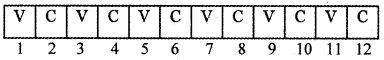(a) Let the first box be filled with a vowel. There are six alternate places available for 6 vowels.
∴ Number of ways of filling 6 vowels in the alternative six boxes is $$\frac{6 !}{2 ! \times 3 !}$$

Remaining 6 boxes can be filled with the 6 consonants. Number of ways of filling the 6 consonants in the remaining 6 boxes is $$\frac{6 !}{2 !}$$

Total number of ways = $$\frac{6 !}{2 ! \times 3 !} \times \frac{6 !}{2 !}$$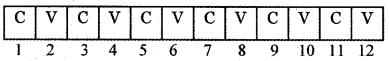(b) Let the first box be filled with a consonant. There six alternate places available for 6 consonants.
∴ Number of ways of filling 6 consonants in the six alternate boxes is $$\frac{6 !}{2 !}$$
Remaining 6 boxes can be filled with the 6 vowels
∴ Number of ways of filling the 6 vowels in the remaining 6 boxes is $$\frac{6 !}{2 ! \times 3 !}$$
Total number of ways = $$\frac{6 !}{2 !} \times \frac{6 !}{2 ! \times 3 !}$$
∴ Total number of strings formed by using the letters of the word INTERMEDIATE, if the vowels and consants are alternative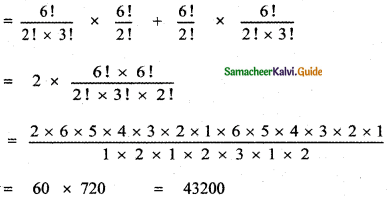(ii) All the vowels are together.
Take all the vowels as 1 unit.
Remaining consonants = 6
Total number of units for arrangement = 7
Number of arrangements = $$\frac{7 !}{2 !}$$
(Since in the consonant’s T’ s repeated twice)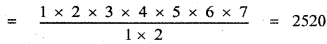Among the vowels, the number of arrangements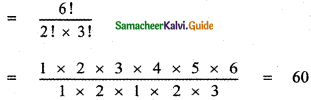∴ Total number of arrangements = 2520 × 60
= 151200

(iii) Vowels are never together :
Total number of arrangements using all theNumber of arrangements where all the vowels are together = 151200
Number of arrangements where all vowels are never together = Total number of arrangements – number of arrangements where the vowels are together = 19958400 – 151200 = 19807200(iv) No two vowels are together:
same as (i)
The number of strings using the letters of the word INTERMEDIATE if no two vowels are together is 43,200.

Question 15.
Each of the digits 1,1,2,3,3 and 4 is written on a separate card. The six cards are then laid out in a row to form a 6 digit number.
(i) How many distinct 6-digit numbers are there?
(i) How many distinct 6-digit numbers are there?
Given numbers are 1, 1, 2, 3, 3, 4
Here 1 occurs twice, 3 occurs twice.
∴ Number of distinct 6 – digit numbers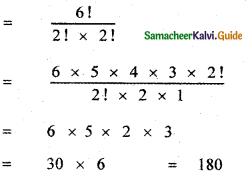(ii) How many of these 6-digit numbers are even?
The given numbers are 1, 1, 2, 3, 3, 4In order to get even 6 – digit numbers, the unit place must be filled by the digits 2 or 4. Therefore, the unit place can be filled in 2 ways using the digits 2 or 4. In the remaining 5 digits (excluding the digit placed in the unit place 2 or 4) 1 occurs 2 times, 3 occurs 2 times.
∴ The number of ways of filling other places using
the remaining 5 digit is = $$\frac{5 !}{2 ! \times 2 !}$$
∴ Number of distinct 6 – digit numbers = $$\frac{5 !}{2 ! \times 2 !} \times 2$$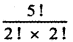= 5 × 4 × 3
= 60(iii) How many of these 6 – digit numbers are divisible by 4?
In order to get the 6 – digit number divisible by 4, the last two digits must be divisible by 4
∴ The last two digits should be 12 or 24 or 32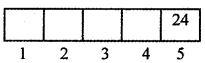Let the last box be filled with 24. The remaining 4 boxes can be filled with the remaining digits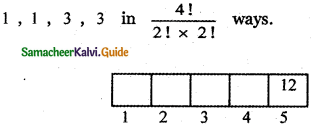Let the last box be filled with 12. The remaining 4 boxes can be filled with the remaining digits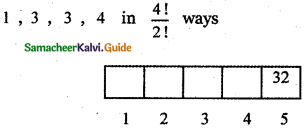Let the last box be filled with 32. The remaining 4 boxes can be filled with the remaining digits
The total number of 6 digit numbers which are divisible by 4 is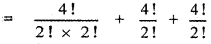= 6 + 12 + 12 = 30
∴ Required number of 6 – digit numbers = 30

Question 16.
If the letters of the word GARDEN are permuted in all possible ways and the strings thus formed are arranged in the dictionary order, then find the ranks of the words
(i) GARDEN
(ii) DANGER.
(i) GARDEN
The letters of the word arranged in the dictionary order is
A, D, E, G, N, R
Total number of letters = 6
The number of words begins with A = 5!
The number of words begins with D = 5!
The number of words begins with E = 5!
The number of words beginning with G = 5!
(But one of these words is GARDEN)
The number of words beginning with GAD = 3!
The number of words beginning with GAE = 3!
The number of words beginning with GAN = 3!
There are 3 ! words beginning with GAR one of these words is GARDEN. The first word beginning with GAR is the word GARDEN.
∴ The rank of the word GARDEN 3 × 120 + 3 × 6 + 1 = 360 + 18 + 1 = 379(ii) DANGER
The dictionary order of the letters of the given word is A, D, E, G, N, R
In the dictionary order of words which begin with A, comes first. If we fill the first place with A, the remaining 5 letters can be arranged in 5! ways. Proceeding like this
Number of words beginning with D = 5! = 120
Number of words beginning with DAE = 3! = 6
Number of words beginning with DAG = 3! = 6
Number of words beginning with DANE = 2! = 2
Number of words beginning with DANGE = 1! = 1
(which is the word DANGER)
∴ The rank of the word DANGER = 120 + 6 + 6 + 2 + 1 = 135

Question 17.
Find the number of strings that can be made using all letters of the word THING. If these words are written in dictionary order, what will be the 85th string?
The given word is THING
Arranging the letters of the word in the dictionary order, we have G, H, I, N, T
The number of strings that can be made using all the letters T, H, I, N, G of the word THING is = 5! = 120
The number of words beginning with G = 4!
The number of words beginning with H = 4!
The number of words beginning with I = 4!
Number of words so far formed = 4! + 4! + 4!
= 24 + 24 + 24 = 72 words
As the required word is in the 85th position, the required word must begin with N Number of words beginning with NG = 3!
A number of words beginning with NH = 3!
Total number of words so far formed
= 72 + 3! + 3!
= 72 + 6 + 6
= 84 words
The next string is the required string. It should begin with NI and its first word beginning with NI which is NIGHT
∴ 85th strings are NIGHTQuestion 18.
If the letters of the word FUNNY are permuted in all possible ways and the strings thus formed are arranged in the dictionary order, find the rank of the word FUNNY.
The given word is FUNNY
To find the rank of the word FUNNY, write down the letters of the word FUNNY. Other than F in alphabetical order N, N, U, Y
Number of words beginning with F = $$\frac{4 !}{2 !}$$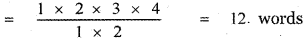Among these words, the number of words beginning with FN = 3! = 1 × 2 × 3 = 6words
(Treating FN as one unit, the remaining 3 letters can be arranged in 3 ! ways)
The number of words beginning with FU is = $$\frac{3 !}{2 !}$$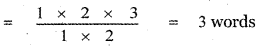Among these three words, FUNNY is the first word, hence among the twelve words beginning with F, FUNNY appears as the 7th word.

Question 19.
Find the sum of all 4 – digit numbers that can be formed using digits 1,2,3,4 and 5 repetitions not allowed?
The given numbers are 1, 2 , 3 , 4 , 5
The total number of arrangements. Using the digits 1, 2, 3, 4 and 5 taking 4 at a time is 5 P4
= 5 × 4 × 3 × 2 = 120
∴ 120 four-digit numbers can be formed using the given 5 digits without repetition. To find the sum of these numbers, we will find the sum of digits at unit’s, ten’s, hundred’s and thousand’s place in all these 120 numbers.

Consider the digit in unit’s place. In all these numbers, each of these digits 1, 2 , 3 , 4, 5 occurs 120
in $$\frac{120}{5}$$ = 24 times in the units place.
∴ The sum of the digits at unit’s place
= 24(1 + 2 + 3 + 4 + 5)
= 24 × 15 = 360
Similarly sum of the digit’s at ten’s place = 360
Sum of the digit’s at hundred’s place = 360
Sum of the digit’s at thousand’s place = 360
∴ Sum of all four digit numbers formed using the digit’s 1 , 2 , 3 , 4 , 5
= 360 × 10° + 360 × 101 + 360 × 102 + 360 × 103
= 360 (10° + 101 + 102 + 103)
= 360 (1 + 10 + 100 + 1000)
= 360 × 1111
= 3,99,960Question 20.
Find the sum of all 4 – digit numbers that can be formed using digits 0,2,5,7,8 without repetition.
The given digits are 0, 2, 5, 7, 8The first box can be filled in 4 ways using the digits 2, 5, 7, 8 ( excluding 0). The second box can be filled in 4 ways using the digits 0, 2, 5, 7, 8 excluding the digit placed in the first box. The third box can be filled in 3 ways using the digits 0, 2, 5, 7, 8 excluding the digits placed in the first two boxes. The fourth box can be filled in 2 ways using the digits 0, 2, 5, 7, 8 excluding the digits placed in the first three boxes.
∴ Total number of 4-digit numbers = 4 × 4 × 3 × 2 = 96
To find the sum of all these four-digit numbers.Fix the number 0 in the list box (4). With the remaining numbers 2, 5, 7, 8, box – 3 can be filled in 4 ways, box – 2 can be filled in 3 ways, and box – 1 can be filled in 2 ways.
∴ Total number of 4 digit numbers ending with 0 is = 4 × 3 × 2 = 24 numbersFix the number 2 in the last box -4. With the remaining digits 0, 5, 7, 8. Box -l can be filled in 3 ways excluding the digit 0. Box – 2 can be filled in 3 ways using the digits 0, 5, 7, 8 excluding the digit placed in a box – 1. Box – 3 can be filled in 2 ways using the digits 0, 5, 7, 8 excluding the digits placed in box-1 and box-2.
∴ Total number of 4 – digit numbers ending with the digit 2 = 3 × 3 × 2 = 18 numbers
Similarly, Total numbers of 4 – digit numbers ending with the digit 5 = 18 numbers
Total number of 4 – digit numbers ending with the digit 7 = 18 numbers
Total number of 4 – digit numbers ending with the digit 8 = 18 numbers
∴ Total for unit place = (24 × 0) + (18 × 2) + (18× 5) + ( 18 × 7) +( 18 × 8)
= 18 × (2+ 5 + 7 + 8)
= 18 × 22 = 396
∴ Sum of the digits at the unit place = 396
Similarly Sum of the digits at ten’s place = 396
Sum of the digit’s at hundred’s place = 396
Sum of the digit’s at thousand’s place = 396
∴ Sum of all four digit numbers formed using the digits 0, 2, 5, 7, 8
= 396 × 10° + 396 × 101 + 396 × 102 + 396 × 103
= 396 × ( 10° + 101 + 102 + 103)
= 396 × ( 1 + 10 + 100 + 1000)
= 396 × 1111 = 571956